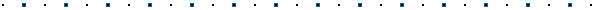#Written by Robert Dunlop Microsoft DirectX MVPNote: This article has been updated for DirectX 9 to accommodate changes in the D3DXComputeBoundingSphere() function.
See DirectX 9 version here## Introduction

Frequently I have received requests for functions that will allow a mesh to be rescaled to fit within specific bounds and/or centered at origin, so I decided to take some time to publish source code to accomplish this task.  It is often necessary to do this when dealing with arbitrary content such as in a mesh viewer, or as a means to verify the validity of imported meshes that may be arbitrarily scaled or offset from origin.  In that context it may also be useful for rescaling mesh geometry so that it can be saved back to a geometry file properly scaled for later use.

## Normalizing a Mesh

Rather than scaling the mesh by a fixed amount, the first function that I am going to show here "normalizes" a mesh - that is, it scales the mesh based on the dimensions of the mesh, so that it will fit inside of a bounding sphere of a specified size.  Normalization typically refers to scaling to unit (1.0) dimensions, but in the case of our function we will allow you to specify the final dimensions of the bounding sphere.

Before we can write our mesh normalization function, we will first need a couple of supporting functions, which will allow us to measure the mesh and to apply scaling and offset.

### Measuring the Mesh

Our first function will allow us to compute the bounding sphere of the mesh, by wrapping the D3DXComputeBoundingSphere() function:

```HRESULT CalcBounds(ID3DXMesh *pMesh, D3DXVECTOR3 *vCenter, float *radius)
{
BYTE *ptr=NULL;
HRESULT hr;

// return failure if no mesh pointer provided
if (!pMesh)
return D3DERR_INVALIDCALL;

// get the face count
DWORD numVerts=pMesh->GetNumVertices();

// get the FVF flags
DWORD fvf=pMesh->GetFVF();  // See DX9 Version

// lock the vertex buffer
if (FAILED(hr=pMesh->LockVertexBuffer(0,&ptr)))

// return on failure
return hr;

// compute bounding sphere
if (FAILED(hr=D3DXComputeBoundingSphere((D3DXVECTOR3 *) ptr,
numVerts,
fvf,  // See DX9 Version
// return on failure
return hr;

// unlock the vertex buffer
if (FAILED(hr=pMesh->UnlockVertexBuffer()))

// return on failure
return hr;

// return success to caller
return S_OK;
}```

### Scaling the Mesh

Our next function allows us to scale and offset the vertices of a mesh:

```HRESULT ScaleMesh(ID3DXMesh *pMesh, float scale, D3DXVECTOR3 *offset=NULL)
{
BYTE *ptr=NULL;
HRESULT hr;
D3DXVECTOR3 vOff;

// return failure if no mesh pointer set
if (!pMesh)
return D3DERR_INVALIDCALL;

// select default or specified offset vector
if (offset)
vOff=*offset;
else
vOff=D3DXVECTOR3(0.0f,0.0f,0.0f);

// get the face count
DWORD numVerts=pMesh->GetNumVertices();

// get the FVF flags
DWORD fvf=pMesh->GetFVF();

// calculate vertex size
DWORD vertSize=D3DXGetFVFVertexSize(fvf);

// lock the vertex buffer
if (FAILED(hr=pMesh->LockVertexBuffer(0,&ptr)))

// return on failure
return hr;

// loop through the vertices
for (DWORD i=0;i<numVerts;i++) {

// get pointer to location
D3DXVECTOR3 *vPtr=(D3DXVECTOR3 *) ptr;

// scale the vertex
*vPtr+=vOff;
vPtr->x*=scale;
vPtr->y*=scale;
vPtr->z*=scale;

// increment pointer to next vertex
ptr+=vertSize;
}

// unlock the vertex buffer
if (FAILED(hr=pMesh->UnlockVertexBuffer()))

// return on failure
return hr;

// return success to caller
return S_OK;
}```

### Our Normalization Function

Finally, we get to our normalization function, which will utilize the two functions we saw above:

1. First, it will find the bounding sphere of the mesh, as a radius and center.
2. Next, it will calculate a scaling factor based on the radius calculated and the desired mesh size.
3. If centering of the mesh is requested, the center will be negated for use as an offset.
4. The scaling function we wrote will then be called with the calculated scaling factor and offset.
```HRESULT NormalizeMesh(ID3DXMesh *pMesh, float scaleTo=1.0f, BOOL bCenter=TRUE)
{
D3DXVECTOR3 vCenter;
HRESULT hr;

// calculate bounds of mesh
return hr;

// calculate scaling factor

// calculate offset if centering requested
D3DXVECTOR3 vOff;
if (bCenter)
vOff=-vCenter;
else
vOff=D3DXVECTOR3(0.0f,0.0f,0.0f);

// scale and offset mesh
return ScaleMesh(pMesh,scale,&vOff);
}```This site, created by DirectX MVP Robert Dunlop and aided by the work of other volunteers, provides a free on-line resource for DirectX programmers.

Special thanks to WWW.MVPS.ORG, for providing a permanent home for this site.

#####Visitors Since 1/1/2000:Last updated: 07/26/05.